ON THEREMIN SENSITIVITY

by Fred Nachbaur

September, 1997; rev. October, 2000

1) Introduction:

Having experimented with theremins for many years now, and gone through the whole gamut of empirical approaches to theremin design, I thought it was time to do a theoretical analysis on that elusive quality referred to as theremin "sensitivity."

By sensitivity I mean essentially: how much of a change in pitch results from a given change in hand position? The same criterion could of course be applied to the volume controller of the theremin, but in this discussion I will refer only to the pitch aspect.

Sensitivity can be compared with to "scale" of a string instrument. A bass viol, for instance, would have a very low sensitivity compared to a 1/4-size violin. In other words, all other things being equal, a semitone change on a bass requires a much greater hand movement than would a semitone change on a violin.

Similarly, there have been many theremin designs over the years, with widely varying degrees of sensitivity. To start our analysis of some of these designs, we have to recognize that it is the change in capacitance between the hand and the pitch antenna that causes a change in pitch. We also have to define some sort of standard of measurement. I propose that sensitivity be defined in kilohertz per picofarad. In other words, by how many kilohertz will the pitch change for a one picofarad change in hand-to-antenna capacitance. This results in easily manageable numbers, that range (depending on design) from less than 0.2 to well over 3.0.

2) Analysing Sensitivity:

Let's start with the basics. All theremin designs (well, okay; almost all) use the LC resonant circuit (L = inductance, as of a coil; C = capacitance) as the frequency-defining elements. Such a circuit is at the heart of the oscillators used in most theremins; the rest of the circuitry provides the positive feedback required to sustain oscillation.

Such LC circuits resonate at the frequency at which the inductive reactance of the coil [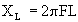] equals the capacitive reactance of the capacitor [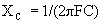]. Setting these two equations equal to each other and solving for F gives the well-known resonance formula:Or, combining constants and scaling for direct use of microhenries and picofarads, we get:

Frequency:where F is in kilohertz, L is in microhenries (µH), and C is in picofarads (pF).

It should be noted that the value for C is the total value of all elements in parallel, including fixed and variable "tank" capacitors, stray circuit capacitances, parasitic antenna capacitance, and of course the all-important hand-to-antenna capacitance. The largest component will usually be the "tank" capacitances, consisting usually of a fixed capacitor (on the order of 100 to 1000 pF), often in parallel with a variable capacitor used for tuning. The parasitic antenna capacitance will usually be on the order of 20 to 50 pF, depending on the geometry and size of the antenna. Stray circuit capacitances are usually minimal, on the order of a few picofarads. The additional hand-to-antenna capacitance is usually the smallest component of the total capacitance, contributing from zero (at an "infinite" distance from the antenna) to only a few picofarads very close to the antenna.

Incidentally, the resonance formula can be used to "back-calculate" the inductance in vintage theremin designs, knowing the tank capacitance and frequency of oscillation. Re-arranging the formula above, we get: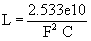(L in µH, F in kHz, C in pF)

For instance, the hand-drawn schematic of "Clara Rockmore's Theremin" shows a 620 pF fixed capacitor in parallel with a 43 pF variable cap and another little unmarked trim cap in the pitch oscillator tank. We can estimate parasitic antenna capacitance at about 50 pF, since she was known to use large rod-type antennas. The grid capacitance of the 27 tube was on the order of 10 pF, so we can estimate that the total tank capacitance was probably on the order of 750 pF. Unfortunately, the circuit gives no indication of inductance, or even of frequency of oscillation.

However, we can take a clue from the liner notes of "The Art of the Theremin," in which it is stated that the oscillating frequency was on the order of a surprisingly low 170 kHz. Plugging these values into our formula for L, we get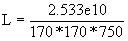= 1170 µH (or 1.17 mH)

The same technique was used to derive an approximate value for the coils used in Doug Forbes' tube design, given his report that the oscillator runs at about 700 kHz. with the capacitor plates at half-mesh.

Back to the issue of sensitivity. Another way of writing our resonance formula is: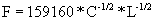Taking the partial derivative with respect to C gives us a direct expression of theremin sensitivity, in kilohertz per picofarad:

Sensitivity: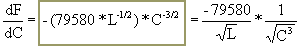Since we took a partial derivative on C, assuming L to be constant, the quantity in the brackets can be lumped together as a constant. Note that the minus sign indicates that frequency decreases with increasing capacitance.

To put the result into words, sensitivity is inversely proportional to the square root of tank capacitance cubed.

Similarly, we can take a partial relative to L, and achieve a similar result; we only have to interchange L and C in the above relationships.

3) Results:

The easiest way to play with this is using a spreadsheet such as Lotus 1-2-3, Excell, or the spreadsheet option in any version of Microsoft Works. You can enter colums for L and C for inputting constants, and columns for F and dF/dC for inputting the appropriate formulas. You can then experiment to your heart's content with various values for L and C, getting immediate reports of center-frequency (F) and sensitivity (dF/dC). You can download the worksheet in 1-2-3 format or in Excel format by right-clicking on the link and selecting "Save Link As..." or "Save Target As...".

The results of such plug 'n' play experimentation using a few known theremin designs is also shown below:

 Design description Induct.L uH Tank C pF Center F kHz dF/dC kHz/pF Clara Rockmore 1165 750 170.266 -0.114 SWTP ("TECI") max L 300 410 453.803 -0.553 SWTP ("TECI") min L 150 410 641.775 -0.783 Theremax max L 350 120 776.597 -3.236 Theremax min L 180 120 1082.913 -4.512 Doug Forbes C=150 500 150 581.446 -1.938 Doug Forbes C=100 500 100 711.763 -3.559 "Theoretical" 1 200 200 796.178 -1.990 "Theoretical" 2 100 400 796.178 -0.995

As you can see, there is a wide range of sensitivity from the lowest (Clara Rockmore) to the highest (Theremax at minimum inductance). The two designs with adjustable inductors (coils) are shown for both extremes of inductance. This demonstrates the dependance on inductance, as predicted by the partial derivative dF/dL. On such designs, you can experiment with different sensitivities, depending on where you set the coils. Similarly, Doug Forbes' design allows a large range of adjustment on the tank capacitor, so sensitivity is shown at two settings (150 pF and 100 pF total).

Two "theoretical" entries are shown also. Both have the same center frequency, but use different ratios of L and C. As you can see, increasing C (while decreasing L to maintain the same resonant frequency) causes a decrease in sensitivity dF/dC.

The general conclusions are that increasing the center frequency (by decreasing inductance or capacitance or both) increases sensitivity. Furthermore, for a given frequency, we can increase sensitivity by maximizing L and minimizing C.

This of course only addresses the electronic aspect of sensitivity. The mechanical geometry of the antenna and even the size of the player's hand can have some effect. However, experience has shown that these effects are small compared with the electronic aspects.

So, more sensitivity is better, right? Not necessarily. It is interesting to note that the original theremin designs had a very low sensitivity compared to the more modern approaches. This means that comparatively large hand motions were required to effect a given pitch change. On the other hand, it means that such older designs are much less "touchy", and therefore a lot easier to play. In my opinion we're making an already difficult instrument even more difficult, even impossible, by increasing sensitivity to the point of absurdity.

4) Variance of Capacitance:

I'll finish with a brief discussion on hand capacitance. The relationship between distance from the antenna and capacitance approximately follows an inverse-square-law. This means that as one approaches the antenna at a constant rate, the capacitance increases very slowly at first, then increases faster and faster as the hand gets closer to the antenna. Very close to the antenna, the capacitance increases astronomically, making the range within an inch or so from the antenna virtually unusable except for "special effects."

This effect is partially offset by the nature of the LC tuned circuit, the frequency of which depends on the inverse square-root of capacitance. This is the main reason, I believe, why oscillators based on a single pole (only one reactive component, i.e. capacitance) never took hold for theremin use. I, and probably many others, have experimented with RC oscillators in an effort to get rid of those pesky coils; even the ordinary NE555 timer can be used for this purpose. However, in such circuits the oscillating frequency is inversely proportional to capacitance, rather than square-root of capacitance, and the "square-law" effect is correspondingly a lot worse. Another way of looking at this is that sensitivity (dF/dC) of the RC circuits is proportional to 1/C2 instead of 1/C1.5 in the case of the LC circuit.

Lest anyone think that this means we're stuck with coils, don't despair. I'm presently working up a coil-less design that behaves exactly like the LC circuits traditionally used. The trick is to use a "virtual inductor" using a high-speed op-amp to simulate a coil. Similarly, varactor diodes can be used instead of mechanical trimmer capacitors to put a modern twist on an old idea, and allow for greater flexibility and improved stability.

As a final note, choice of sensitivity depends a great deal on your playing style. If you want more accurate pitch control, especially at higher frequencies, choose a design with a low sensitivity. If you work mainly with lower frequencies, use a higher sensitivity; you won't need as much motion to effect a given pitch change.